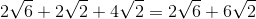# ISEE Upper Level Math : How to find the perimeter of a right triangle

## Example Questions

### Example Question #1 : How To Find The Perimeter Of A Right Triangle

One angle of a right triangle measures 45, and the hypotenuse length is 6 centimeters. Give the perimeter of the triangle.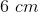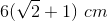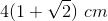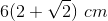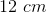Explanation:

This triangle has two angles of 45 and 90 degrees, so the third angle must measure 45 degrees; this is therefore an isosceles right triangle.

By the Pythagorean Theorem, the square of the hypotenuse is equal to the sum of the squares of the other two sides.

Let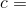hypotenuse and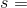side length.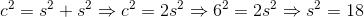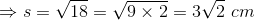We can now find the perimeter since we have all three sides: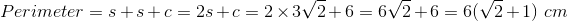### Example Question #2 : How To Find The Perimeter Of A Right Triangle

One leg of a right triangle measures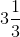; the hypotenuse measures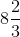. What is the perimeter of the triangle?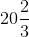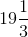Explanation:

The length of the second leg can be calculated using the Pythagorean Theorem. Set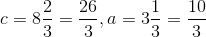: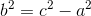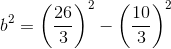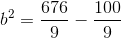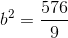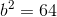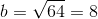Add the three sidelengths to get the perimeter: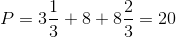### Example Question #3 : How To Find The Perimeter Of A Right Triangle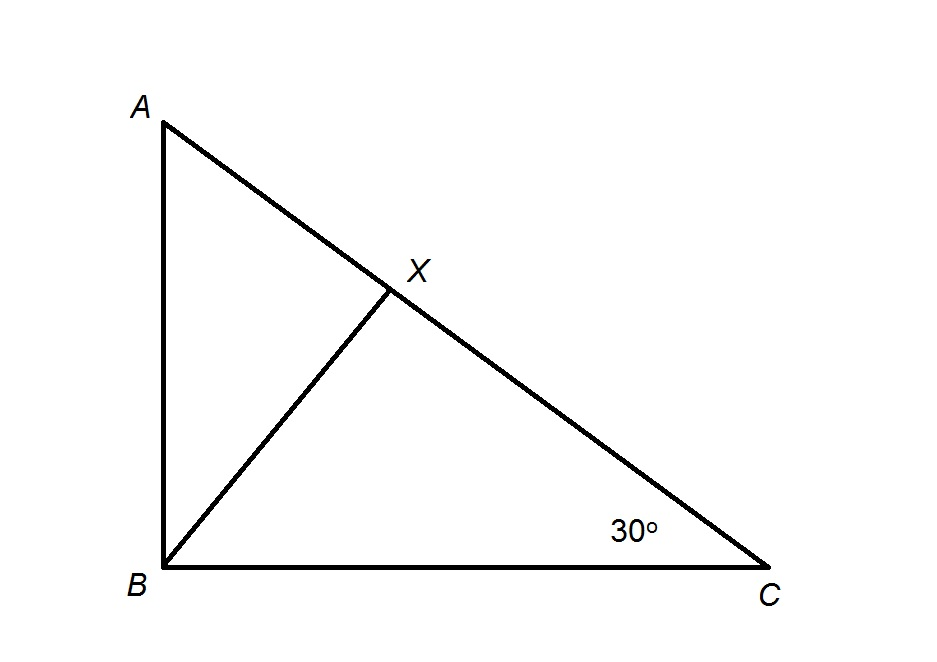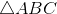is a right triangle with altitude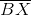. If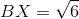, give the perimeter of.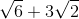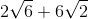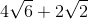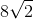Explanation:

An altitude of a right triangle, which here is, divides the triangle into two triangles similar to each other and to the large triangle. Therefore, their corresponding angles are equal.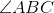is right - and has degree measure 90 - and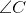has degree measure 30, so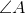has degree measure 60, making the triangle a 30-60-90 triangle. Therefore, the smaller triangles are as well.is the short leg of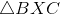, so the hypotenuse,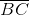, has twice the length of that leg, or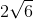is the long leg of; short leg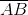has as its length the length ofdivided by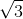, or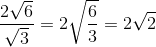.

The hypotenuse of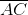, has as its length twice the length of short leg, which is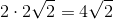.

Add the lengths of the sides of: